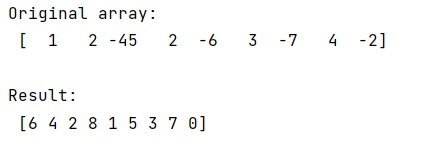# Find the index of the k smallest values of a NumPy array

Learn, how to find the index of the k smallest values of a NumPy array in Python?
Submitted by Pranit Sharma, on January 08, 2023

NumPy is an abbreviated form of Numerical Python. It is used for different types of scientific operations in python. Numpy is a vast library in python which is used for almost every kind of scientific or mathematical operation. It is itself an array which is a collection of various methods and functions for processing the arrays.

## NumPy Array - Finding the index of the k smallest values

Suppose we are given a numpy array and also a specific integer value up to which we need to find the smallest values of this array.

For this purpose, we will use numpy.argpartition() method that states that the kth element is in a sorted position and all smaller elements will be moved before it. Thus the first k elements will be the k-smallest elements.

Let us understand with the help of an example,

## Python code to find the index of the k smallest values of a NumPy array

```# Import numpy
import numpy as np

# Import pandas
import pandas as pd

# Creating an array
arr = np.array([1,2,-45,2,-6,3,-7,4,-2])

# Display array
print("Original array:\n",arr,"\n")

# Defining value for k
k = 3

# Finding index of kth smallest values
ind = np.argpartition(arr, k)

# Display result
print("Result:\n",ind,"\n")
```

Output: